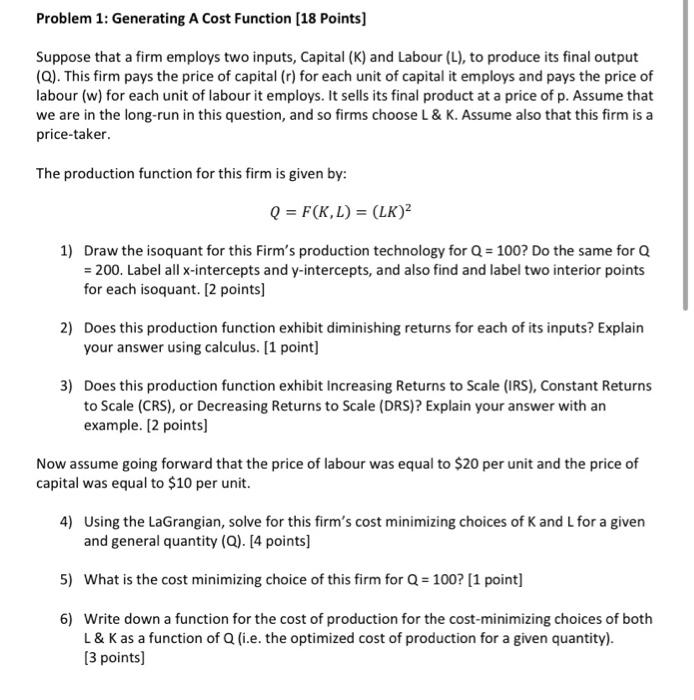Home / Expert Answers / Economics / q3-4-suppose-that-a-firm-employs-two-inputs-capital-k-and-labour-l-to-produce-its-final-output-pa141

# (Solved): q3,4 Suppose that a firm employs two inputs, Capital (K) and Labour (L), to produce its final output ...

q3,4Suppose that a firm employs two inputs, Capital (K) and Labour (L), to produce its final output (Q). This firm pays the price of capital ( for each unit of capital it employs and pays the price of labour (w) for each unit of labour it employs. It sells its final product at a price of . Assume that we are in the long-run in this question, and so firms choose L \& K. Assume also that this firm is a price-taker. The production function for this firm is given by: 1) Draw the isoquant for this Firm's production technology for ? Do the same for . Label all -intercepts and -intercepts, and also find and label two interior points for each isoquant. [2 points] 2) Does this production function exhibit diminishing returns for each of its inputs? Explain your answer using calculus. [ 1 point] 3) Does this production function exhibit Increasing Returns to Scale (IRS), Constant Returns to Scale (CRS), or Decreasing Returns to Scale (DRS)? Explain your answer with an example. [2 points] Now assume going forward that the price of labour was equal to per unit and the price of capital was equal to per unit. 4) Using the LaGrangian, solve for this firm's cost minimizing choices of and for a given and general quantity (Q). [4 points] 5) What is the cost minimizing choice of this firm for ? [ 1 point] 6) Write down a function for the cost of production for the cost-minimizing choices of both as a function of (i.e. the optimized cost of production for a given quantity). [3 points]

We have an Answer from Expert